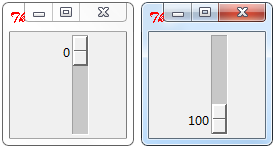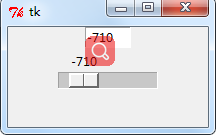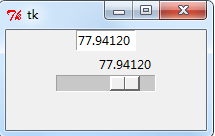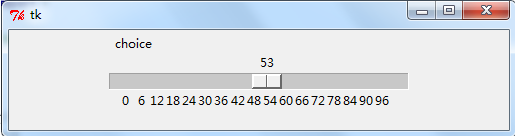Tkinter 中的 Scale 控制元件是一種可供使用者通過拖動指示器改變變數值的控制元件. 這種控制元件可以水平放置, 也可以豎直放置.

from Tkinter import *
root=Tk()

Scale(root).pack()

root.mainloop()from Tkinter import *
root=Tk()
root.geometry('200x200')
Scale(root,from_=-1000,to=1000,resolution=10,orient=HORIZONTAL).pack()

root.mainloop()
from_ ,設定最大值；to ,設定最小值；resolution ,設定步距值；orient = HORIZONTAL #設定水平方向

Scale繫結變數並顯示在Entry中
from Tkinter import *
root=Tk()
root.geometry('200x100')

v=IntVar()
Entry(root,textvariable=v,width=6).pack()
# variable = v #繫結變數
Scale(root,from_=-1000,to=1000,resolution=10,orient=HORIZONTAL,variable=v).pack()

root.mainloop()from Tkinter import *
root=Tk()
root.geometry('200x100')

v=IntVar()

def CallOn(text):
return text
Entry(root,textvariable=str(CallOn(v)),width=6).pack()

# variable = v #繫結變數
Scale(root,from_=-1000,to=1000,resolution=10,orient=HORIZONTAL,variable=v,command=CallOn).pack()

root.mainloop()

from Tkinter import *
root=Tk()
root.geometry('200x100')

v=IntVar()

def CallOn(text):
return text
Entry(root,textvariable=str(CallOn(v)),width=8).pack()

# variable = v #繫結變數
Scale(root,from_=0,to=100,resolution=0.0001,orient=HORIZONTAL,variable=v,command=CallOn,digits=8).pack()

root.mainloop()from Tkinter import *
root=Tk()
root.geometry('200x100')

v=IntVar()

def CallOn(text):
return text
Entry(root,textvariable=str(CallOn(v)),width=8).pack()

# variable = v #繫結變數
Scale(root,label='choice',from_=0,to=100,resolution=0.0001,orient=HORIZONTAL,variable=v,command=CallOn,digits=8).pack()

root.mainloop()

label設定的值會顯示在水平Scale的上方，用於提示資訊

set（）與get（）
from Tkinter import *
root=Tk()
root.geometry('200x100')

v=IntVar()

def CallOn(text):
print S.get()

S=Scale(root,label='choice',from_=0,to=100,resolution=0.0001,orient=HORIZONTAL,variable=v,digits=8,command=CallOn)

#初始值
S.set(50)
S.pack()
root.mainloop()

tickinterval 選項被設定為一個數值, 拖動時的選擇值將會變為該數值的倍數

from Tkinter import *
root=Tk()
root.geometry('500x100')

v=IntVar()

def CallOn(text):
print S.get()

S=Scale(root,label='choice',from_=0,to=100,tickinterval=6,orient=HORIZONTAL,variable=v,command=CallOn,length=300)

#初始值
S.set(50)
S.pack()
root.mainloop()